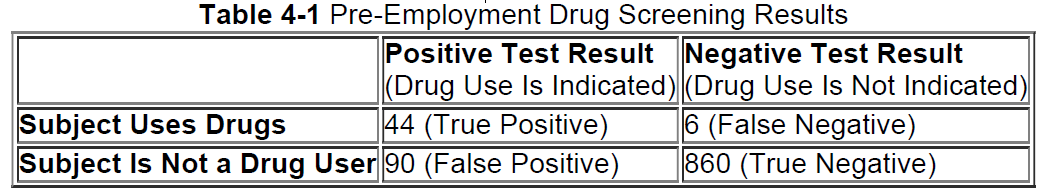×
Get Full Access to Elementary Statistics - 12 Edition - Chapter 4.4 - Problem 15bsc
Get Full Access to Elementary Statistics - 12 Edition - Chapter 4.4 - Problem 15bsc

×

# Answer: Pre-Employment Drug Screening. In Exercise, useISBN: 9780321836960 18

## Solution for problem 15BSC Chapter 4.4

Elementary Statistics | 12th Edition

• Textbook Solutions
• 2901 Step-by-step solutions solved by professors and subject experts
• Get 24/7 help from StudySoup virtual teaching assistantsElementary Statistics | 12th Edition

4 5 1 287 Reviews
19
4
Problem 15BSC

Pre-Employment Drug Screening. In Exercises 13–16, use the test results summarized in Table 41, reproduced here. Consider an event to be “unlikely” if its probability is 0.05 or less.Pre-Employment Drug Screening If 3 of the 1000 test subjects are randomly selected, find the probability that they all had correct test results (either true positive or true negative). Is such an event unlikely?

a. Assume that the 3 selections are made with replacement.

b. Assume that the 3 selections are made without replacement.

Step-by-Step Solution:
Step 1 of 3

Solution  15BSC

=0.739

Step 2 of 3

Step 3 of 3

##### ISBN: 9780321836960

The answer to “?Pre-Employment Drug Screening. In Exercises 13–16, use the test results summarized in Table 41, reproduced here. Consider an event to be “unlikely” if its probability is 0.05 or less. Pre-Employment Drug Screening If 3 of the 1000 test subjects are randomly selected, find the probability that they all had correct test results (either true positive or true negative). Is such an event unlikely?a. Assume that the 3 selections are made with replacement.b. Assume that the 3 selections are made without replacement.” is broken down into a number of easy to follow steps, and 81 words. Elementary Statistics was written by and is associated to the ISBN: 9780321836960. Since the solution to 15BSC from 4.4 chapter was answered, more than 458 students have viewed the full step-by-step answer. This full solution covers the following key subjects: test, employment, true, assume, drug. This expansive textbook survival guide covers 121 chapters, and 3629 solutions. The full step-by-step solution to problem: 15BSC from chapter: 4.4 was answered by , our top Statistics solution expert on 03/15/17, 10:30PM. This textbook survival guide was created for the textbook: Elementary Statistics, edition: 12.

## Discover and learn what students are asking

Calculus: Early Transcendental Functions : The Natural Logarithmic Function: Integration
?In Exercises 1-26, find the indefinite integral. $$\int \frac{5}{x} d x$$

Calculus: Early Transcendental Functions : Extrema of Functions of Two Variables
?In Exercises 7 - 20, examine the function for relative extrema and saddle points. $$f(x, y)=x^{2}-x y-y^{2}-3 x-y$$

Statistics: Informed Decisions Using Data : Applications of the Normal Distribution
?Explain why P(X ? 220) should be reported as > 70.9999 if X is a normal random variable with mean 100 and standard deviation 15.

Unlock Textbook Solution